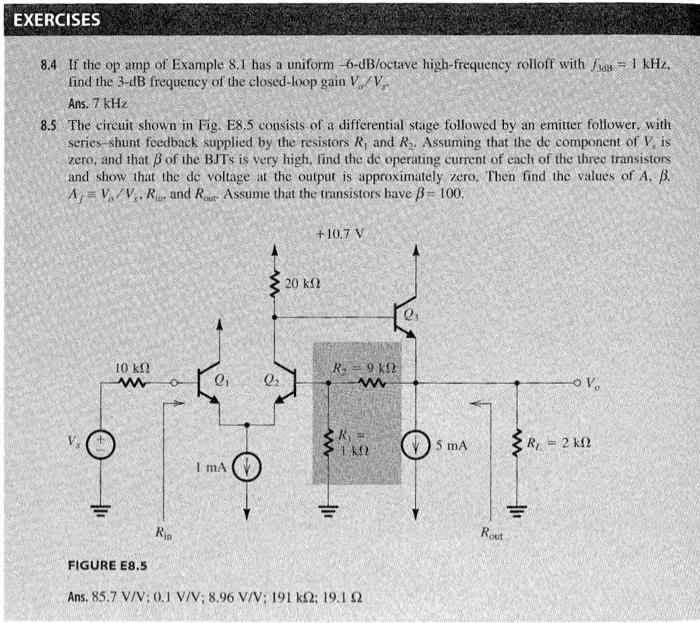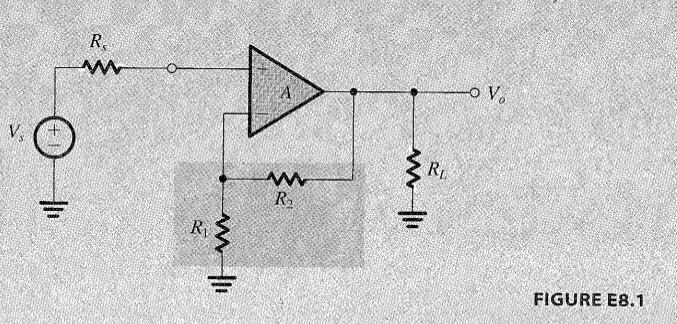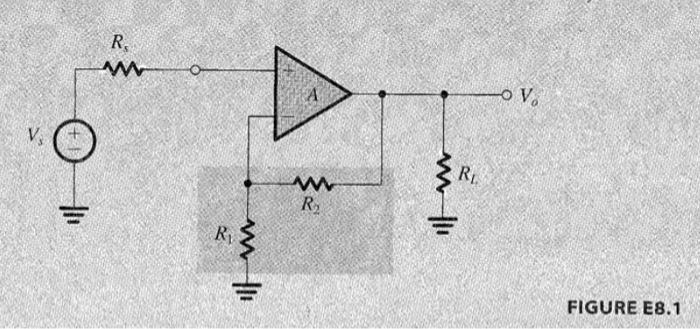# Question Solved1 AnswerEXERCISES 8.4 If the op amp of Example 8.1 has a uniform -6-dB/octave high-frequency rolloff with 7 = 1 kHz. find the 3-dB frequency of the closed-loop gain V/V, Ans. 7 kHz 8.5 The circuit shown in Fig. E8.5 consists of a differential stage followed by an emitter follower, with series-shunt feedback supplied by the resistors R, and R. Assuming that the de component of V, is zero, and that B of the BJTs is very high, find the de operating current of each of the three transistors and show that the de voltage at the output is approximately zero. Then find the values of A. B. A = V/V, R. and Rout. Assume that the transistors have ß- 100. +10.7 V 20 ΚΩ 1Ο ΚΩ w R9K2 02 w OV. O SRI ΙΚΩ SMA -222 w I mA = RO Rout FIGURE E8.5 Ans. 85.7 V/V:0.1 V/V: 8.96 V/V: 191 k12: 19.1 92 R w A -OV V. + RL w R in lli RI w FIGURE E8.1 R w -OV V RL w R in lli w RI FIGURE E8.1XGKU9Z The Asker · Electrical EngineeringTranscribed Image Text: EXERCISES 8.4 If the op amp of Example 8.1 has a uniform -6-dB/octave high-frequency rolloff with 7 = 1 kHz. find the 3-dB frequency of the closed-loop gain V/V, Ans. 7 kHz 8.5 The circuit shown in Fig. E8.5 consists of a differential stage followed by an emitter follower, with series-shunt feedback supplied by the resistors R, and R. Assuming that the de component of V, is zero, and that B of the BJTs is very high, find the de operating current of each of the three transistors and show that the de voltage at the output is approximately zero. Then find the values of A. B. A = V/V, R. and Rout. Assume that the transistors have ß- 100. +10.7 V 20 ΚΩ 1Ο ΚΩ w R9K2 02 w OV. O SRI ΙΚΩ SMA -222 w I mA = RO Rout FIGURE E8.5 Ans. 85.7 V/V:0.1 V/V: 8.96 V/V: 191 k12: 19.1 92 R w A -OV V. + RL w R in lli RI w FIGURE E8.1 R w -OV V RL w R in lli w RI FIGURE E8.1
More
Transcribed Image Text: EXERCISES 8.4 If the op amp of Example 8.1 has a uniform -6-dB/octave high-frequency rolloff with 7 = 1 kHz. find the 3-dB frequency of the closed-loop gain V/V, Ans. 7 kHz 8.5 The circuit shown in Fig. E8.5 consists of a differential stage followed by an emitter follower, with series-shunt feedback supplied by the resistors R, and R. Assuming that the de component of V, is zero, and that B of the BJTs is very high, find the de operating current of each of the three transistors and show that the de voltage at the output is approximately zero. Then find the values of A. B. A = V/V, R. and Rout. Assume that the transistors have ß- 100. +10.7 V 20 ΚΩ 1Ο ΚΩ w R9K2 02 w OV. O SRI ΙΚΩ SMA -222 w I mA = RO Rout FIGURE E8.5 Ans. 85.7 V/V:0.1 V/V: 8.96 V/V: 191 k12: 19.1 92 R w A -OV V. + RL w R in lli RI w FIGURE E8.1 R w -OV V RL w R in lli w RI FIGURE E8.1